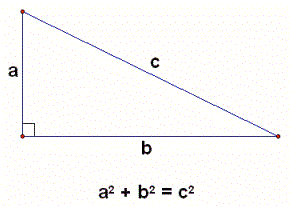# The Pythagorean theorem

The Pythagorean theorem was reportedly formulated by the Greek mathematician and philosopher Pythagoras of Samos in the 6th century BC. It says that the area of the square whose side is the hypotenuse of the triangle is equal to the sum of the areas of the squares whose sides are the two legs of the triangle. If you write it in the form of an equation, it looks like this:

a2 + b2 = c2

In this equation, c represents the length of the hypotenuse, while the legs are represented by a and b.In this form, the Pythagorean theorem enables you to find the length of any side in a right triangle if you know the other two, as well as to check if a triangle is a right triangle. This proves quite useful in solving math problems during education, as well as in a number of real life situations. We are going to illustrate this through these few examples:

Example 1

The length of one side is 9 cm and the length of the other side is 10 cm. Calculate the length of the hypotenuse.

This is a very simple problem. We know the length of two sides of the triangle and to determine the length of the hypotenuse, we just have to insert the lengths we know into the equation.

c2 = a2 + b2

c2 = 92 + 102

c2 = 81 + 100

c2 = 181

c = 13,5 cm

We see now that the missing length of the hypotenuse is 13,5 cm and that is our result. The next assignment will be a bit more complicated.

Example 2

The length of the hypotenuse is 10,6 cm and the length of one of the legs is 5,2 cm. Find the length of the other leg of the triangle.

This is also very simple. Let us name the hypotenuse c and the side we already know – a. The missing side is b. To get b from our equation we need to rearrange it a bit. Like this:

b2 = c2 – a2

The only thing left to do now is to insert the known values into the equation.

b2 = 10,62 – 5,22

b2 = 112,36 – 27,04

b2 = 85,32

b = 9,2 cm

The length of the missing side is 9,2 cm. Now let us take a look at one more example.

Example 3

The length of the hypotenuse is 6,5 cm, the length of leg a is 5,6 cm and the length of leg b is 3,3. Do these sides belong to a right triangle?

As before, this can also be solved easily. We will take the lengths of two sides of the triangle in question, calculate the length of the third using the Pythagorean theorem and check if our result is a match to the length of the hypotenuse of our triangle. We will do it like this:

c2 = 5,62 + 3,32

c2 = 42,25

c = 6,5 cm

As we can see, the length we calculated if identical to the given length of this triangle. This means that the triangle in question is indeed a right triangle.

These are just some of the numerous uses of the Pythagorean theorem. If you wish to practice working with the Pythagorean theorem, please feel free to use the math worksheets below.

## Pythagorean theorem exams for teachers

 Exam Name File Size Downloads Upload date Integers Pythagorean theorem – Integers – very easy 456.4 kB 27192 September 3, 2019 Pythagorean theorem – Integers – easy 590.8 kB 20990 September 3, 2019 Pythagorean theorem – Integers – medium 592.9 kB 31385 September 3, 2019 Pythagorean theorem – Integers – hard 594.5 kB 17708 September 3, 2019 Pythagorean theorem – Integers – very hard 607 kB 13805 September 3, 2019 Decimals Pythagorean theorem – Decimals – very easy 456.4 kB 5394 September 3, 2019 Pythagorean theorem – Decimals – easy 599.4 kB 3994 September 3, 2019 Pythagorean theorem – Decimals – medium 596 kB 6321 September 3, 2019 Pythagorean theorem – Decimals – hard 599.2 kB 5048 September 3, 2019 Pythagorean theorem – Decimals – vey hard 605.9 kB 5761 September 3, 2019

## Pythagorean theorem worksheets for students

 Worksheet Name File Size Downloads Upload date Integers Pythagorean theorem – Integers – Find the missing hypotenuse 3.4 MB 74861 September 3, 2019 Pythagorean theorem – Integers – Find the missing leg 3.4 MB 38539 September 3, 2019 Pythagorean theorem – Integers – Find the missing parameter 3.3 MB 15876 September 3, 2019 Pythagorean theorem – Integers – Right triangle or not 2.8 MB 17926 September 3, 2019 Decimals Pythagorean theorem – Decimals – Find the missing hypotenuse 7.1 MB 7437 September 3, 2019 Pythagorean theorem – Decimals – Find the missing leg 7.2 MB 5632 September 3, 2019 Pythagorean theorem – Decimals – Find the missing parameter 7.1 MB 6246 September 3, 2019 Pythagorean theorem – Decimals – Right triangle or not 7.6 MB 5413 September 3, 2019